Tamilnadu State Board New Syllabus Samacheer Kalvi 11th Physics Guide Pdf Chapter 6 Gravitation Text Book Back Questions and Answers, Notes.

## Tamilnadu Samacheer Kalvi 11th Physics Solutions Chapter 6 Gravitation

### 11th Physics Guide Gravitation Book Back Questions and AnswersI. Multiple choice questions:

Question 1.
The linear momentum and position vector of the planet is perpendicular to each other at:
(a) perihelion and aphelion
(b) at all points
(c) only at perihelion
(d) no point
(a) perihelion and aphelion

Hint:
At aphelion Potential Energy is more and Kinetic energy is less.
At perihelion Potential Energy is less and Kinetic Energy is more.

Question 2.
If the masses of the Earth and Sun suddenly double, the gravitational force between them will:
(a) remain the same
(b) increase 2 times
(c) increase 4 times
(d) decrease 2 times
(c) increase 4 times

Hint: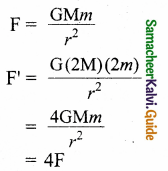Question 3.
A planet moving along an elliptical orbit is closest to the Sun at distance r1 and farthest away at a distance of r2. If v1 and v2 are linear speeds at these points respectively. Then the ratio $$\frac{v_{1}}{v_{2}}$$ is: (NEET 2016)
(a) $$\frac{r_{2}}{r_{1}}$$
(b) ($$\frac{r_{2}}{r_{1}}$$)²
(c) $$\frac{r_{1}}{r_{2}}$$
(d) ($$\frac{r_{1}}{r_{2}}$$)²
(a) $$\frac{r_{2}}{r_{1}}$$

Hint:
v = rw
∴ v ∝ r
$$\frac{v_{1}}{v_{2}}$$ = $$\frac{r_{1}}{r_{2}}$$Question 4.
The time period of a satellite orbiting Earth in a circular orbit is independent of:
(b) The mass of the satellite
(c) Both the mass and radius of the orbit
(d) Neither the mass nor the radius of its orbit
(b) The mass of the satellite
Hint:
T= 2π$$\sqrt{\frac{\mathrm{RE}}{g}}$$
Since there is no mass in the expression, time period is independent of mass.

Question 5.
If the distance between the Earth and Sun were to be doubled from its present value, the number of days in a year would be:
(a) 64.5
(b) 1032
(c) 182.5
(d) 730
(b) 1032Question 6.
According to Kepler’s second law, the radial vector to a planet from the Sun sweeps out equal areas in equal intervals of time. This law is a consequence of:
(a) conservation of linear momentum
(b) conservation of angular momentum
(c) conservation of energy
(d) conservation of kinetic energy
(a) conservation of linear momentum

Hint:
Kepler’s second law is a consequence of conservation angular momentum.

Question 7.
The gravitational potential energy of the Moon with respect to Earth is:
(a) always positive
(b) always negative
(c) can be positive or negative
(d) always zero
(b) always negative

Hint:
Because when two masses come together slowly from infinity work is done on the system.Question 8.
The kinetic energies of a planet in an elliptical orbit about the Sun, at positions A, B and C are KA, KB and Kc respectively. AC is the major axis and SB is perpendicular to AC at the position of the Sun S as shown in the figure. Then: (NEET 2018)(a) KA > KB > KC
(b) KB < KA < KC
(c) KA < KB < KC
(d) KB > KA > KC
(a) KA > KB > KC

Hint:
Kinetic energy depends on the velocity of a planet. Planets travel fast when they are nearer to the sun and slower when they are farther from it.

Question 9.
The work done by the Sun’s gravitational force on the Earth is:
(a) always zero
(b) always positive
(c) can be positive or negative
(d) always negative
(c) can be positive or negative

Question 10.
If the mass and radius of the Earth are both doubled, then the acceleration due to gravity g’:
(a) remains same
(b) y
(c) $$\frac { g }{ 2 }$$
(d) 4 g
(b) y

Hint:
g = $$\frac{\mathrm{GM}}{\mathrm{R}^{2}}$$
M’ = 2M
R’ = 2R
g’ = $$\frac{\mathrm{GM}^{\prime}}{\left(\mathrm{R}^{\prime}\right)^{2}}$$
= $$\frac{G \times 2 M}{(2 R)^{2}}$$
= $$\frac{\mathrm{GM}}{\mathrm{2R}^{2}}$$
= $$\frac { g }{ 2 }$$

Question 11.
The magnitude of the Sun’s gravitational field as experienced by Earth is:
(a) same over the year
(b) decreases in the month of January and increases in the month of July
(c) decreases in the month of July and increases in the month of January
(d) increases during day time and decreases during night time.
(c) decreases in the month of July and increases in the month of January

Hint:
Gravitational field $$\vec { E }$$ = – $$\frac{\mathrm{GM}}{\mathrm{R}^{2}}$$$$\hat{r}$$
It depends on the distance r that vary from January to July.Question 12.
If a person moves from Chennai to Trichy, his weight:
(a) increases
(b) decreases
(c) remains same
(d) increases and then decreases
(a) increases

Hint:
W = mg
g = $$\frac{\mathrm{GM}}{\mathrm{R}^{2}}$$
∴ W = $$\frac{\mathrm{GMm}}{\mathrm{R}^{2}}$$
Weight depends on distance ‘r’.

Question 13.
An object of mass 10 kg is hanging on a spring scale which is attached to the roof of a lift. If the lift is in free fall, the reading in the spring scale is:
(a) 98 N
(b) zero
(c) 49 N
(d) 9.8 N
(b) zero

Hint:
If the lift is free fall then a = g.
∴ N = m(g – g) = 0
∴ The reading will be zero.

Question 14.
If the acceleration due to gravity becomes 4 times of original value, then escape speed:
(a) remains same is directly proportional to the product of
(b) 2 times of original value masses and inversely proportional to square
(c) becomes halved of the distance between the masses.
(d) 4 times of original value 3. Will the angular momentum of a planet be
(b) 2 times of original value masses and inversely proportional to square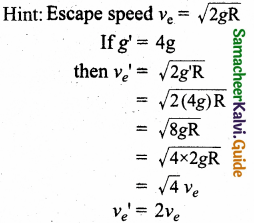Question 15.
The kinetic energy of the satellite orbiting around the Earth is:
(a) equal to potential energy
(b) less than potential energy
(c) greater than kinetic energy
(d) zero
(b) less than potential energy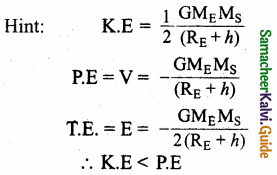Question 1.
State Kepler’s three laws.

1. Law of Orbits: Each planet revolves moves around the Sun in an elliptical orbit with the Sun at one of the foci of the ellipse.
2. Law of area: The radial vector line joining the Sun to a planet sweeps equal areas in equal intervals of time.
3. Law of period: The square of the time period of revolution of a planet around the Sun in its elliptical orbit is directly proportional to the cube of the semi-major axis of the ellipse.

Question 2.
State Newton’s Universal law of gravitation.
The gravitational force between two masses is directly proportional to the product of masses and inversely proportional to square of the distance between the masses.

Question 3.
Will the angular momentum of a planet be conserved? Justify your answer.
The torque experienced by the Earth due to the gravitational force of the Sun is given by,It implies that angular momentum $$\vec { L }$$ is constant vector. Hence L is conserved.

Question 4.
Define the gravitational field. Give its unit.
The gravitational field intensity $$\overrightarrow{\mathrm{E}}_{1}$$ at a point is defined as the gravitational force experienced by unit mass at that point. It’s unit N kg-1.Question 5.
What is meant by superposition of gravitational field?
The total gravitational field at a point due to all the masses is given by the vector sum of the gravitational field due to the individual masses.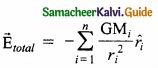Question 6.
Define gravitational potential energy.
Potential energy of a body at a point in a gravitational field is the work done by an external agent in moving the body from infinity to that point.

Question 7.
Is potential energy the property of a single object? Justify.
No, potential energy is not the property of a single system. It is due to the position of the object. Because, potential energy depends on two masses and the distance between them.

Question 8.
Define gravitational potential.
The gravitational potential is defined as the amount of work required to bring unit mass from infinity to that point.

Question 9.
What is the difference between gravitational potential and gravitational potential energy?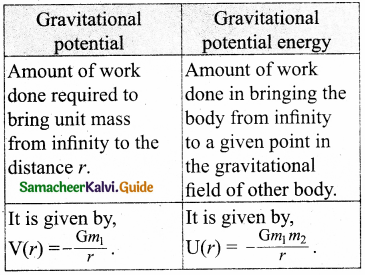Question 10.
What is meant by escape speed in the case j of the Earth?
The escape speed is independent of the direction in which the object is thrown. Irrespective of whether the object is thrown vertically up, radially outwards or tangentially it requires the same initial speed to escape Earth’s gravity force. This can be written as, ve = $$\sqrt{2 g R_{E}}$$.

Question 11.
Why is the energy of a satellite (or any other planet) negative?
The negative sign of the total energy implies that satellite is bound to the Earth’s gravitational force. So it cannot escape from the Earth. At large distances satellite is not bound to Earth. It is completely free from gravitational force of the Earth.Question 12.
What are geostationary and polar satellites?
Geostationary Satellite: It is the satellite which appears at a fixed position and at a definite height to an observer on earth.
Polar Satellite: It is the satellite which revolves in polar orbit around the earth.

Question 13.
Define weight.
The weight is defined as the downward force whose magnitude ‘w ’ is equal to that of upward force that must be applied to the object to hold it at rest or at constant velocity relative to the Earth.

Question 14.
Why is there no lunar eclipse and solar eclipse every month?
If the orbits of the Moon and Earth lie on the same plane, during full Moon of every month, we can observe lunar eclipse. If this is so dining new Moon we can observe solar eclipse. But Moon’s orbit is tilted 5° with respect to Earth’s orbit. Due to this 5° tilt, only during certain periods of the year, the Sun, Earth and Moon align in straight line leading to either lunar eclipse or solar eclipse depending on the alignment.

Question 15.
How will you prove that Earth itself is spinning?
When the position of a star is observed over a night, it appears to move in circular motion about the pole star. This fact proves that Earth itself is spinning.Question 1.
Discuss the important features of the law of gravitation.
(i) Because of inverse dependence on square of the distance the distance between two masses increases, the strength of the force tends to decrease.

(ii) Because Uranus is at larger distance from the Sun compared to the Earth, the planet Uranus experiences less gravitational force from the Sun than the Earth.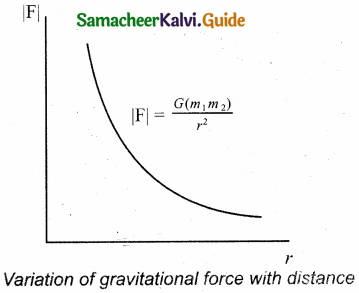(iii) The gravitational forces between two particles always constitute an action- reaction pair. From this, it is known that gravitational force exerted by the Sun on the Earth is always towards the Sun. The reaction-force is exerted by the Earth on the Sun. The direction of reaction is towards Earth.

(iv) The torque experienced by the Earth due to the gravitational force of the Sun is given by,It implies that angular momentum $$\vec { L }$$ is a constant vector. The angular momentum of Earth about the Sun is constant throughout the motion. It is true for all the planets.

(v) The expression $$\vec { F }$$ = $$\frac{\mathrm{GM}_{1} \mathrm{M}_{2}}{r^{2}} \hat{r}$$. Here it was assumed that both Earth and Sun are treated as point masses.

(vi) This assumption is a good approximation because the distance between the two bodies is very much larger than their diameters.

(vii) The force of attraction between a hollow sphere of mass M with uniform density and point mass m kept outside the hollow sphere is calculated by replacing the hollow sphere of mass M as equivalent to a point mass M located at the centre of the hollow sphere.

The force of attraction between the hollow sphere of mass M and point mass ‘m’ can be calculated by considering the hollow sphere also as another point mass. It is shown in the Figure below.

(viii) If we place another object of mass ‘m ’ inside a hollow sphere of mass M as in Figure below, the force experienced by this mass ‘m ’ will be zero.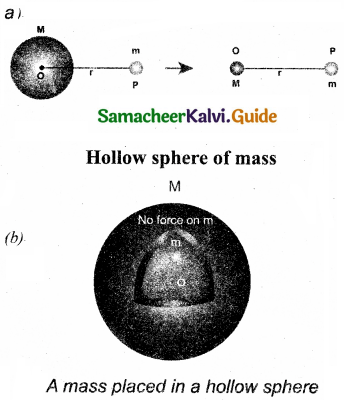(ix) The success of the law of gravitation is that it concludes that the mango that is falling down and the Moon orbiting the Earth are due to the same gravitational force.

Question 2.
Explain how Newton arrived at his law of gravitation from Kepler’s third law.
Newton considered the orbits of the planets as circular. For circular orbit of radius r, the centripetal acceleration towards the centre is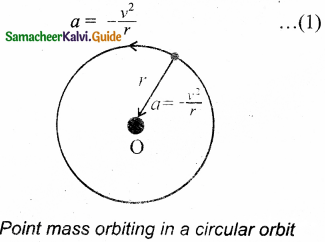Here v is the velocity and r, the distance of the planet from the centre of the orbit.
The velocity in terms of known quantities r and T, is
v = $$\frac { 2πr }{ T }$$              … (2)
Here T is the time period of revolution of the planet. Substituting this value of ‘v’ in equation (1) we get,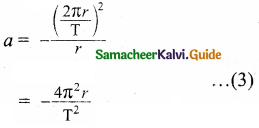Substituting the value of ‘a’ from (3) in Newton’s second law, F = ma where ‘m’ is the mass of the planet.
F = – $$\frac{4 \pi^{2} m r}{\mathrm{r}^{2}}$$ … (4)
From Kepler’s third law,
$$\frac{r^{3}}{\mathrm{~T}^{2}}$$ = k (constant) … (5)
$$\frac{r}{\mathrm{~T}^{2}}$$ = $$\frac{k}{r^{2}}$$ … (6)
By using equation (6) in the force expression, we can arrive at the law of gravitation.
F = – $$\frac{4 \pi^{2} m k}{\mathrm{r}^{2}}$$ … (7)
Here negative sign implies that the force is attractive and it acts towards the centre. He equated the constant 4π²k to GM which turned out to be the law of gravitation.
F = – $$\frac{\mathrm{GMm}}{r^{2}}$$Question 3.
Explain how Newton verified his law of gravitation.
The gravitational force experienced by the apple due to Earth.
F = – $$\frac{\mathrm{GM}_{\mathrm{E}} \mathrm{M}_{\mathrm{A}}}{\mathrm{R}^{2}}$$
MA – Mass of the apple
ME – Mass of the Earth
R – Radius of the Earth.
Equating the above equation with Newton’s second law, we get
MAaA = – $$\frac{\mathrm{GM}_{\mathrm{E}} \mathrm{M}_{\mathrm{A}}}{\mathrm{R}^{2}}$$
Simplifying the above equation we get,
aA = – $$\frac{\mathrm{GM}_{\mathrm{E}}}{\mathrm{R}^{2}}$$
aA is the acceleration of apple that is equal to ‘g’.
Similarly the force experienced by Moon due to Earth is given by
F = – $$\frac{\mathrm{GM}_{\mathrm{E}} \mathrm{Mm}}{\mathrm{R}_{m}^{2}}$$
Rm – distance of the Moon from the Earth.
Mm – Mass of the Moon.
The acceleration experienced by the Moon is given by,
am = – $$\frac{\mathrm{GM}_{\mathrm{E}}}{\mathrm{R}_{m}^{2}}$$
The ratio between the apple’s acceleration to Moon’s acceleration is given by
$$\frac{a_{\mathrm{A}}}{a_{m}}=\frac{\mathrm{R}_{m}^{2}}{\mathrm{R}^{2}}$$
From the Hipparchrus measurement, the distance to the Moon is 60 times that of Earth radius.
Rm = 60R.
$$\frac{a_{\mathrm{A}}}{a_{m}}=\frac{(60 \mathrm{R})^{2}}{\mathrm{R}^{2}}$$
The apple’s acceleration is 3600 times the acceleration of the Moon.
The same result was obtained by Newton using his gravitational formula.

Question 4.
Derive the expression for gravitational potential energy.
Consider two masses m1 and m2 are initially separated by a distance r’. m1 is assumed to be fixed in its position.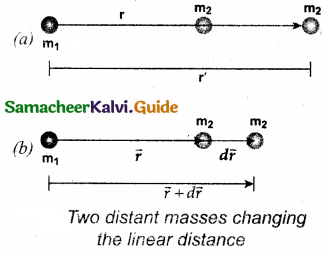To move the mass m2 through an infinitesimal displacement $$\vec { dr }$$ from $$\vec { r }$$ to $$\vec { r }$$ + $$\vec { dr }$$ (shown in the Figure above), work has to be done externally. This infinitesimal work is given by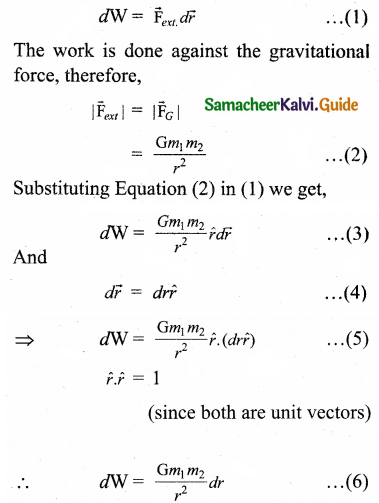Hence the total work done for displacing the particle from r’ to r isQuestion 5.
Prove that at points near the surface of the Earth, the gravitational potential energy of the object is U = mgh.
Let us consider the Earth and mass system, with r, the distance between the mass ‘nr and the Earth’s centre. Then the gravitational potential energy,
U = $$\frac{\mathrm{GM}_{e} m}{r}$$ … (1)
Here r = Re + h, where Re is the radius of the Earth, h is the height above the Earth’s surface.
U = – G $$\frac{\mathrm{M}_{e} m}{\left(\mathrm{R}_{e}+h\right)}$$ … (2)
If h << Re, equation (2) can be modified as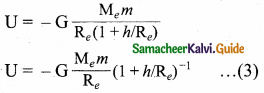By using Binomial expansion and neglecting the higher order terms, we get
U = – $$\mathrm{G} \frac{\mathrm{M}_{e} m}{\mathrm{R}_{e}}\left(1-\frac{h}{\mathrm{R}_{e}}\right)$$ … (4)
We know that, for a mass m on the Earth’s surface,
G$$\frac{\mathrm{M}_{e} m}{\mathrm{R}_{e}}$$ = mgRe … (5)
Substituting equation (5) in (4) we get,
U = mgRe + mgh … (6)
In the equation (6) the first term can be omitted or taken to zero. Thus it can be stated that the gravitational potential energy stored in the particle of mass m at a height h from the surface of the Earth is U = mgh.Question 6.
Explain in detail the idea of weightlessness using lift as an example.
Freely falling bodies experience gravitational force only. As they fall freely, they are not in contact with any surface. When a lift falls with downward acceleration a = g, the person inside the lift is in the state of free fall or weightlessness. The normal force acting on the object is zero. In this case, the downward acceleration is equal to the acceleration due to the gravity of the Earth. i.e., (a = g). From equation,
N = m(g – a)
we get,
a= g
∴ N = m(g – g) = 0.
This is called the state of weightlessness.

Example: When the lift falls (when the lift wire cuts) with downward acceleration a = g, the person inside the elevator is in the state of weightlessness or free fall.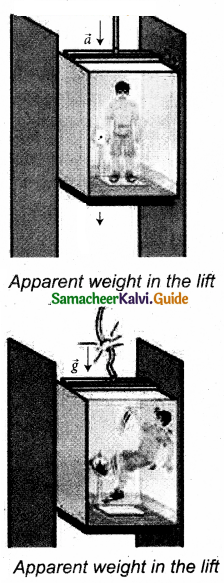Question 7.
Derive an expression for escape speed.
Consider an object of mass M on the surface of the Earth. When it is thrown up with an initial speed vi the initial total energy of the object is
Ei = $$\frac { 1 }{ 2 }$$Mvi² – $$\frac{\mathrm{GMM}_{\mathrm{E}}}{\mathrm{R}_{\mathrm{E}}}$$ … (1)

Where, ME is the mass of the Earth and RE – the radius of the Earth. The term $$\frac{\mathrm{GMM}_{\mathrm{E}}}{\mathrm{R}_{\mathrm{E}}}$$ is the potential energy of the mass M.

When the object reaches a height far away from Earth and as approaching infinity, the gravitational potential energy becomes zero [U(∞) = 0] and the kinetic energy becomes zero as well. Hence the final total energy of the object becomes zero. It holds good for minimum energy and for minimum speed to escape. Otherwise Kinetic energy can be non-zero.
Ef = 0
According to the law of energy conservation,
Ei = Ef … (2)
Substituting (1) in (2) we get,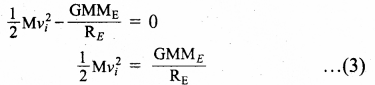The minimum speed required by an object to escape Earth’s gravitational field is escape speed. Hence vi is replaced ve. i.e.,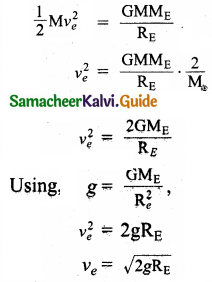Question 8.
Explain the variation of ‘q’ with latitude.
An object is on the surface of the Earth, experiences a centrifugal force that depends on the latitude of the object on Earth. If the Earth were not spinning, the force on the object would have been mg. An additional centrifugal force due to spinning of the Earth, is experienced by the object.This centrifugal force is given by mω²R’.
R’= R cos λ,
where λ is the latitude. The component of centrifugal acceleration experienced by the object in the direction opposite to acceleration due to gravity isQuestion 9.
Explain the variation of ‘g’ with altitude.
Let us consider an object of mass m at a height h from the surface of the Earth. Acceleration experienced by the object due to Earth is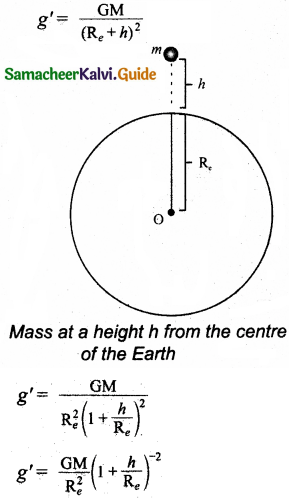If h << Re
Using Binomial expansion and taking the terms upto first order.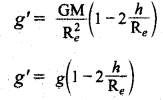We find that g’

Question 10.
Explain the variation of g with depth from the Earth’s surface.
Let us consider a particle of mass m which is in a deep mine on the Earth. Let the depth of the mine as d. To calculate g’ at a depth d, consider the following points.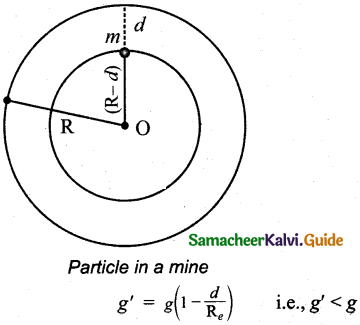The part of the Earth that is above the radius (Re – d) do not contribute to the acceleration. The result is proved earlier and is given as GM
g’ = $$\frac{\mathrm{GM}^{\prime}}{\left(\mathrm{R}_{e}-d\right)^{2}}$$
M’ is the mass of the Earth of radius (Re – d)
Assuming the density of Earth p to be constant,
ρ = $$\frac { M }{ V }$$
Where M is the mass of the Earth and V its volume, Thus,Here also g'< g. As depth increases, g’ decreases.

Question 11.
Derive the time period of satellite orbiting the Earth.
The distance covered by the satellite during one rotation in its orbit is equal to 2π(RE + h) and time taken for it is the time period, T. Then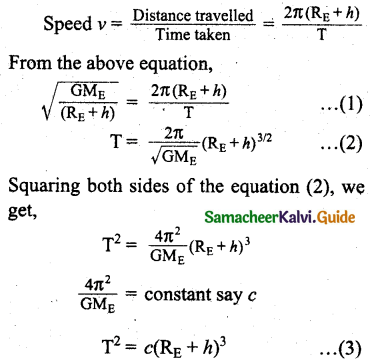Equation (3) implies that a satellite orbiting the Earth has the same relation between time and distance as that of Kepler’s law of planetary motion. For a satellite orbiting near the surface of the Earth, h is negligible compared to the radius of the Earth RE. Then,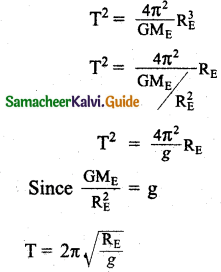Question 12.
Derive an expression for energy of satellite.
The total energy of the satellite is the sum of its kinetic energy and the gravitational potential energy. The potential energy of the satellite is,
U = – $$\frac{\mathrm{GM}_{\mathrm{S}} \mathrm{M}_{\mathrm{E}}}{\left(\mathrm{R}_{\mathrm{E}}+h\right)}$$ … (1)
Here Ms – Mass of the satellite, ME – Mass of the Earth, RE – Radius of the Earth.
The Kinetic energy of the satellite is
K.E = $$\frac { 1 }{ 2 }$$Ms … (2)
Here v is the orbital speed of the satellite and is equal to
v = $$\sqrt{\frac{\mathrm{GM}_{\mathrm{E}}}{\left(\mathrm{R}_{\mathrm{E}}+h\right)}}$$ … (3)
Substituting the value of v in (2), the kinetic energy of the satellite becomes,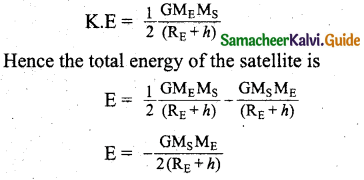Question 13.
Explain in detail thegeo-stationary and polar satellites.
Geo-stationary satellite is the satellite which revolves around the Earth with same speed and in the same direction as the Earth rotates.
RE + h = $$\left(\frac{\mathrm{GM}_{\mathrm{E}} \mathrm{T}^{2}}{4 \pi^{2}}\right)$$ … (1)
By substituting the values for the time period, mass and radius of the Earth, in equation (1) h turns out to be 36,000 km. Such satellites are called “geo – stationary satellites”, since they appear to be stationary when seen from Earth. INSAT group of satellites that are basically geo – stationary satellites used for the purpose of telecommunication.

A Polar satellite covers a small strip of area from pole to pole during one revolution. In the next revolution it covers a different strip of area. Since the Earth would have moved by a small angle. In this way polar satellites cover the entire surface area of the Earth.Question 14.
Explain how geocentric theory is replaced by heliocentric theory using the idea of retrograde motion of planets.
(i) During the observation of motion of the planets it is in the night sky by naked eyes over a period of a few months, it can be seen that the planets move eastwards and reverse their motion for a while and return to eastward motion again. This is called “retrograde motion” of planets.

(ii) Ptolemy introduced the concept of “epicycle” in his geocentric model. To explain this retrograde motion, according to this theory, while the planet orbited the Earth, it also underwent another circular motion termed as “epicycle”. A combination of epicycle and circular motion around the Earth gave rise to retrograde motion of the planets with respect to Earth.

(iii) But Ptolemy’s model became more and more complex as every planet was found to undergo retrograde motion.

(iv) According to heliocentric model, the Sun is at the centre of the solar system and all planets orbited the Sun. The retrograde motion of planets ‘with respect to Earth is due to the relative motion of the planet with respect to Earth. The retrograde motion from the heliocentric point of view.The Earth orbits around the Sun faster than Mars. Due to the relative motion between Mars and Earth, Mars appears to move backwards from July to October. In the same way the retrograde motion of all other planets was explained successfully by the Copemicus model. The heliocentric model slowly replaced the geocentric model, because of its simplicity.

Question 15.
Explain in detail the Eratosthenes method of finding the radius of Earth.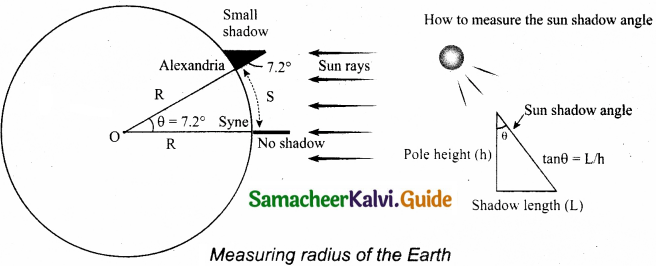Eratosthenes observed that during noon time of summer solstice the Sun’s rays cast no shadow in the city Syne. It was located 500 miles away from Alexandria. At the same day and same time he found that in Alexandria the Sun’s rays made an angle 7.2 degree with local vertical as shown in the figure. He realized that this difference of 7.2 degree was due to the curvature of the Earth.
The angle 7.2 degree is equivalent to $$\frac { 1 }{ 8 }$$ radian. So θ = $$\frac { 1 }{ 8 }$$ rad.
If S is the length of the arc between the cities of Syne and Alexandria, and if R is radius of Earth, then,
S = Rθ = 500 miles
So, the radius of the Earth.
R = $$\frac { 500 }{ θ }$$ miles
R = 500 $$\frac { miles }{ 1/8 }$$
R = 4000 miles
1 mile is equal to 1.609 km. So, he measured the radius of the Earth to be equal to R = 6436km, which is amazingly close to the correct value of 6378km.

Question 16.
Describe the measurement of Earth’s shadow (umbra) radius during total lunar eclipse.It is possible to measure the radius of the shadow of the Earth at the point where the Moon crosses. When the Moon exits from the umbra shadow, it appears red in color. As soon as the Moon exits from the umbra shadow, it appears in a crescent shape.
By knowing the apparent radii of the Earth’s umbra shadow and the Moon, the ratio of these radii can be calculated.
The apparent radius of the Moon = Rm = 5.15 cm.
The ratio $$\frac{\mathrm{R}_{s}}{\mathrm{R}_{m}}$$ ≈ 2.56
Rs = 2.56 x Rm
The radius of Moon Rm = 1737km
Rs = 2.56 x 1737 km ≅ 4446km.

IV. Conceptual Questions:

Question 1.
According to Kepler, planet move in
(a) Circular orbits around the Sun
(b) Elliptical orbits around the Sim with Sun at the exact centre
(c) Straight lines with constant velocity
(d) Elliptical orbits around the Sun with Sun at one of its foci.
(d) Elliptical orbits around the Sun with Sun at one of its foci.

Question 2.
The work done by Sun on Earth in one year will be:
(a) zero
(b) non-zero
(c) positive
(d) negative
(d) negativeQuestion 3.
Rockets are launched in an eastward direction to take advantage of …..
(a) the clear sky on the eastern side
(b) Earth’s rotation
(c) the thinner atmosphere on this side
(d) Earth’s tilt
(b) Earth’s rotation
Hint:
Because of Earth rotation from west to east direction.

Question 4.
If a comet suddenly hits the Moon and imparts energy which is more than the total energy of the Moon, what will happen?
A comet with small velocity and high mass, doesn’t trigger the moon much. It just makes a circular-shaped impact. The moon is ment for the protection for life on earth and to attain stability for the earth rotation. But a comet with large mass and with large velocity may destroy the moon completely or its impact makes the moon, go out of its orbit.

Question 5.
If the Earth’s pull on the Moon suddenly disappears, what will happen to the Moon?
Earth’s pull is,
F = $$\frac{\mathrm{GM}_{\mathrm{E}} \mathrm{M}_{m}}{\mathrm{R}_{m}^{2}}$$
The moon will move away with largest distance of approach. Motion of the moon would cease.

Question 6.
Define orbital velocity.
Orbital velocity is the velocity required to put the satellite into its orbit around the earth.

Question 7.
A student was asked the question why are there summer and winter for us’? He replied as ‘since Earth is orbiting in an elliptical orbit, when the Earth is very far away from the Sun(aphelion) there will be winter when the Earth is nearer to the Sun(perihelion) there will be winter’. Is this answer correct? if not, what is the correct explanation for the occurrence of summer and winter?
Early astronomers proved that Earth is spherical in shape by looking at the shape of the shadow cast by Earth on the Moon during a lunar eclipse.

Question 8.
The following photographs are taken from the recent lunar eclipse which occurred on January 31, 20.18. Is it possible to prove that Earth is a sphere from these photographs?From the photograph, the apparent radii of the Earth’s umbra shadow and the radius of the Moon, and the apparent radius of Earth can be calculated. By looking at the shape of the shadow caused by Earth on the Moon during lunar eclipse. We can confirm that Earth is spherical in shape.V. Numerical Problems:

Question 1.
An unknown planet orbits the Sun with distance twice the semi-major axis distance of the Earth’s orbit, if the Earth’s time period is T1 what is the time period of this unknown planet.
Given: a1 = 2a1
To find: T2 = ??
Formula: T² ∝ a³ => T ∝ (a)3/2
T2 ∝ (2a1)3/2
∝ (2)3/2 (a1)3/2
T2 ∝ 2$$\sqrt{2}$$ T1
∴ T2 = (2/$$\sqrt{2}$$)T1.

Question 2.
Assume that you are in another solar system and provided with the set of data given below consisting of the planet’s semi major axes and time periods. Can you infer the relation connecting semi-major axis and time period?Time period T² ∝ a³
T1 = 2; a1 = 8
a1 = 2² x 2 = 2T1 (T1 = 2)
a2 = 3² x 2 = 2T2² (T2 = 3)
a3 = 4² x 2 = 2T3² (T3 = 4)
a4 = 5² x 2 = 2T4² (T4 = 5)
a5 = 6² x 2 = 2T5² (T5 = 6)
∴ a ∝ 2T²

Question 3.
If the masses and mutual distance between the two objects are doubled, what is the change in the gravitational force between them?
Given:
m1‘ = 2m1
m2‘ = 2m2
r1 = 2r
Formula:There is no change in the gravitational force.Question 4.
Two bodies of masses m and Am are placed at a distance r. Calculate the gravitational potential at a point on the line joining them where the gravitational field is zero.
Given: m1 = m
m2 = 4m
distance = r
To find: V = ??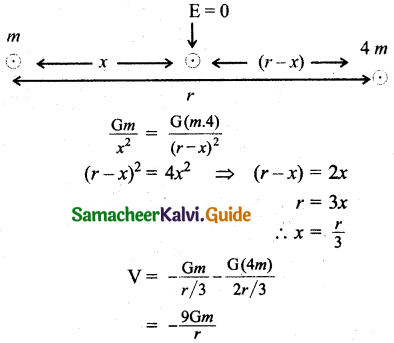Question 5.
If the ratio of the orbital distance of two planets $$\frac{d_{1}}{d_{2}}$$ = 2, what is the ratio of gravitational field experienced by these two planets?
Given: $$\frac{d_{1}}{d_{2}}$$ = 2
Formula:
Gravitational field,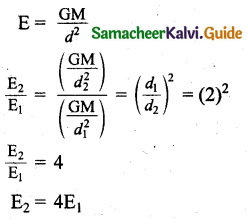Question 6.
The Moon lo orbits Jupiter once in 1.769 days. The orbital radius of the Moon lo is 421700 km. Calculate the mass of Jupiter?Question 7.
If the angular momentum of a planet is given by $$\vec{L}$$ = 5t²$$\hat{i}$$-6t$$\hat{f}$$+3$$\hat{k}$$. What is the torque experienced by the planet? Will the torque be in the same direction as that of the angular momentum?Question 8.
Four particles, each of mass M and equidistant from each other, move along a circle of radius R under the action of their mutual gravitational attraction. Calculate the speed of each particle.
Force acting on a particleSince particle, moving circular path experience centripetal force,Question 9.
Suppose unknowingly you wrote the universal gravitational constant value as G = 6.67 x 1011 instead of the correct value G = 6.67 x 10-11, what is the acceleration due to gravity g’ for this incorrect G? According to this new acceleration due to gravity, what will be your weight W?
Incorrect value of G = 6.67 x 1011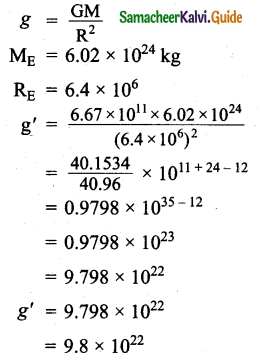If mass m = 1
then weight W’ = mg’- 9.8 x 1022
W’ = 9.8 x 1022W

Question 10.
Calculate the gravitational field at point O due to three masses m1, m2 and m3 whose positions are given by the following figure. If the masses m1 and m2 are equal what is the change in a gravitational field at point O?The distance of masses m1 and m2 from the origin in the X-axis is ‘a’ and m3 in y-axis also ‘a’Question 11.
What is the gravitational potential energy of the Earth and Sun? The Earth to Sun distance is around 150 million km. The mass of the Earth is 5.9 x 1024 kg and the mass of the Sun is 1.9 x 1030 kg.
Given: r = 150 million km
r = 150 x 106 km
= 150 x 109m
Me = 5.9 x 1024 kg
Ms = 1.9 x 1030kg
To find: Potential energy = ??
Formula: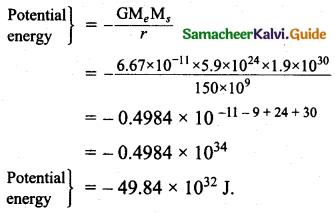Question 12.
Earth revolves around the Sun at 30 km s1. Calculate the kinetic energy of the Earth. In the previous example, you calculated the potential energy of the Earth. What is the total energy of the Earth in that case? Is the total energy positive? Give reasons.
Given: V=30km/sFormula:
$$\left.\begin{array}{l} \text { Kinetic } \\ \text { energy(K.E) } \end{array}\right\}$$ = $$\frac { 1 }{ 2 }$$mv²
= $$\frac { 1 }{ 2 }$$ x 5.9 x 1024 x 30 x 30 x 1032
= $$\frac { 1 }{ 2 }$$ x 5.9 x 3 x 3 x 1032
K.E = 26.55 x 1032 J.
∴ T.E = K.E + P.E
T.E = 26.55 x 1032 – 49.84 x 1032
T.E = – 23.29 x 1032J.
Total energy is negative. It implies Earth is bounded with Sun.Question 13.
An object is thrown from Earth in such a way that it reaches a point at infinity with non-zero kinetic energy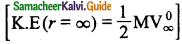, with what velocity should the object be thrown from Earth?
From the law of conservation of energyQuestion 14.
Suppose we go 200 km above and below the surface of the Earth, what are the g values at these two points? In which case, is the value of g small?
Given: depth (d) = height(A) = 200 km
We know that R – 6400 km
Formula:Question 15.
ω²R = ( $$\frac { 1 }{ 2 }$$ )²R
= $$\left(\frac{2 \times 3.14}{24 \times 60 \times 60}\right)^{2}$$ x 6400 x 10³